Courses

# Relational Algebra,Tuple Calculus And SQL (Advance Level)- 1

## 15 Questions MCQ Test Question Bank for GATE Computer Science Engineering | Relational Algebra,Tuple Calculus And SQL (Advance Level)- 1

Description
This mock test of Relational Algebra,Tuple Calculus And SQL (Advance Level)- 1 for Computer Science Engineering (CSE) helps you for every Computer Science Engineering (CSE) entrance exam. This contains 15 Multiple Choice Questions for Computer Science Engineering (CSE) Relational Algebra,Tuple Calculus And SQL (Advance Level)- 1 (mcq) to study with solutions a complete question bank. The solved questions answers in this Relational Algebra,Tuple Calculus And SQL (Advance Level)- 1 quiz give you a good mix of easy questions and tough questions. Computer Science Engineering (CSE) students definitely take this Relational Algebra,Tuple Calculus And SQL (Advance Level)- 1 exercise for a better result in the exam. You can find other Relational Algebra,Tuple Calculus And SQL (Advance Level)- 1 extra questions, long questions & short questions for Computer Science Engineering (CSE) on EduRev as well by searching above.
QUESTION: 1

### Given relations R(w, x) and S(y, z), the result of SELECT DISTINCT w,x FROM R, S Is guaranteed to be same as R, if

Solution:

The given query
SELECT DISTINCT W, X
FROM R, S
Is guaranteed to be same as R, if R has no duplicates and ‘S’ is non-empty.
Since, if R is having a duplicates, then the tuples selected by SELECT operation of the R and the given query will not be same also if ‘S’ is empty then the given query outputs null.

QUESTION: 2

### Branch-scheme = (Branch - name, assets, branch- city) Customer-scheme = (Customer-name, street, customer- city) Deposit-scheme = (Branch-name, account-number, customer-name, balance) Borrow-scheme = (Branch-name, loan-number, customer-name, amount) Client-scheme = (Customer-name, banker-name) Using relational algebra, the query that finds customers who have a balance of over 1000 is

Solution:

From the relation DEPOSIT first we have to select the those entries in which balance is over 1000. This can be obtained by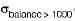(Deposit), Now, we have to select those customers name who have balance over 1000 which can be obtained by simply projecting customer name in above subquery.

QUESTION: 3

### ​Branch-scheme = (Branch - name, assets, branch- city) Customer-scheme = (Customer-name, street, customer- city) Deposit-scheme = (Branch-name, account-number, customer-name, balance) Borrow-scheme = (Branch-name, loan-number, customer-name, amount) Client-scheme = (Customer-name, banker-name) Which of the following queries finds the clients of banker Agassi and the city they live in?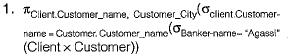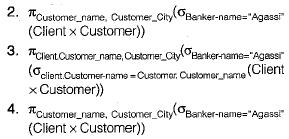Solution:

Clients of Bankers Agassi can be obtained by using the relation client_scheme while the corresponding customer_city can be obtained -using customer_scheme.
Hence cross product of client and customer must be taken. This can be achieved in two ways.
(i) First taking the Bankers Agassi clients and then checking their name in customer relation and projecting their customer city.
(ii) First taking all the customers and matching them in customer_scheme, then finding out the customers who have the Agassi as the Banker, finally projecting the customer_city of the respective customers.

QUESTION: 4

​Branch-scheme = (Branch - name, assets, branch- city)
Customer-scheme = (Customer-name, street, customer- city)
Deposit-scheme = (Branch-name, account-number, customer-name, balance)
Borrow-scheme = (Branch-name, loan-number, customer-name, amount)
Client-scheme = (Customer-name, banker-name)

Which of the following tuple relational calculus finds all customers who have a loan amount of more than 1200?

Solution:

We have to find all customers in the relation Borrow, this can be achieved by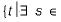borrow (t [custome _name ])=s [customer_name]
The s here is bound variable and the condition of checking Ioan-amount more than 1200 is to be checked with each customer name condition.

QUESTION: 5

Branch-scheme = (Branch - name, assets, branch- city)
Customer-scheme = (Customer-name, street, customer- city)
Deposit-scheme = (Branch-name, account-number, customer-name, balance)
Borrow-scheme = (Branch-name, loan-number, customer-name, amount)
Client-scheme = (Customer-name, banker-name)
Which of the following Domain relational calculus finds all customers who have a loan amount of over 1200?

Solution:

All customers who have a lone amount of over 1200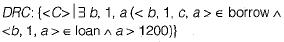QUESTION: 6

Consider the set of relations given below and the SQL query that follows:
Students: (Roll_number, Name, date_of_birth)
Courses: (Course_number, Course_name, Instructor)
SELECT DISTINCT Name
RolLnumber
ANDCourse.lnstructor = Korth
Which of the following sets is computed by the above query?

Solution:

The WHERE condition checks for the Grade = ‘A' and matching of course-number in the courses and Grades, where the instructor must be ‘Korth’. Overall it checks for students to whom ‘Korth’ has instructed and they have received Grade = ‘A in atleast one of the courses taught by ‘Korth’.

QUESTION: 7

Consider the following relational schema pertaining to a students database.
Enroll: (rollno. courseno. coursename) Where the primary keys are shown underlined. The number of tuples in the Student and the Enroll tables are 120 and 8 respectively. What are the maximum and the minimum number of tuples that can be present in (Student * Enroll), where * denotes natural join?

Solution:

The maximum number of tuples results when each of the 120 students enrolls for each of the 8. Courses, giving 120 x 8 = 960 tuples . The minimum number of tuples results when all the 120 students enroll for the same course, giving 120 x 1 - 120 tuples.

QUESTION: 8

The employee information in a company is stored in the relation. Assume name is the primary key:
Employee: (name, sex, salary, deptName)
Consider the SQL query;
SELECT deptName
FROM Employee
WHERE sex = M
GROUP By deptName
HAVING avg(salary) > (SELECT avg(salary)
FROM Employee)
It returns the names of the departments in which the average salary.

Solution:

The given query first calculates the average salary from the relation Employee, then it selects the department name where employees who have average salary more than the average salary of the company.

QUESTION: 9

Choose the correct statements:

Solution:

Relation algebra is procedural query language while relational calculus is non-procedural query language.

QUESTION: 10

The SQL expression :
SELECT distinct 7.branch_name
FROM branch T, branch S
WHERE T.assets >S.assets and S.branch_city = "PONDICHERRY”
Finds the names of

Solution:

The condition T. assets > S. assets and S. branch_city = “PONDICHERRY” checks for the all those branch-name who have greater assets than any branch located in Pondicherry. The keyword distinct removes the duplicacy of branch_name satisfy the given condition.

QUESTION: 11

If P and Q are predicates and P is the relational algebra expression, then which of the following equivalence are valid?

Solution:

All of the given option valid: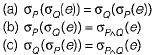They simply find and selects the tuples which are common to both P and Q.

QUESTION: 12

Which of the following query transformation (i.e. replacing the LHS expression by the RHS expression) is incorrect? R1 and R2, are relations, C1 C2 are selection conditions and A1 A2 are attributes of R1.

Solution: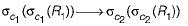The conditions c1 and c2 may not be same.

QUESTION: 13

Consider a following declaration: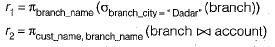Then to find the customers who appears in r2, with every branch name in r1 the query is:

Solution:

Find the customers who appears in r2 with every branch name in r1
So, it is represented by division query: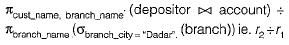QUESTION: 14

With the help of which of the following relations operation set we can perform division on relations?

Solution:

With the help of {π, x, -} we can perform division operation.
Note: Division operator produces a relation R(X) that includes all tuples t[x] in R(z) that appear in R in combination with every tuple from R2y) when Z = X ∪ Y.

QUESTION: 15

Which of the following relational calculas expressions is not safe?

Solution:

A relational calculus expression may contain infinite tuples that are not in the relation, R1.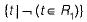Hence, the above expression is not safe.